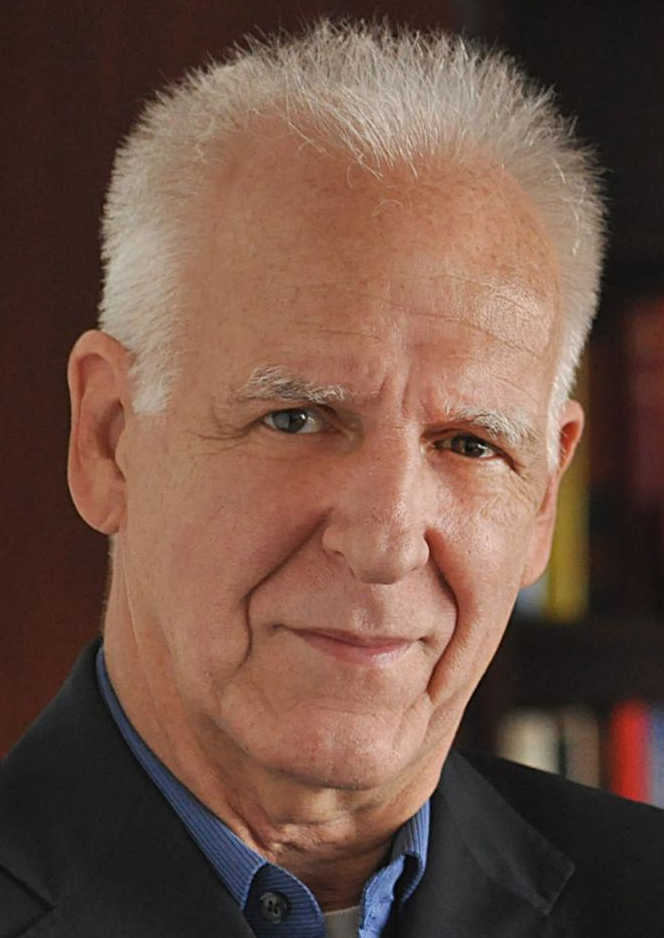## Optimality in Learning

Ronald DeVore
Texas A&M University

Learning an unknown function f from data observations arises in a myriad of application domains. This talk will present the mathematical view of learning. It has two main ingredients:

• a model class assumption which summarizes the totality of information we have about f in addition to the data observations
• a metric which describes how we measure performance of a learning algorithm.

We first mathematically describe optimal recovery which is the best possible performance for a learning algorithm. While optimal recovery provides the ideal benchmark on which to evaluate performance of a numerical learning algorithm, it does not, in and of itself, provide a numerical recipe for learning.

We then turn to the construction of discrete optimization problems whose solution provides a provably near optimal solution to the learning problem. We compare these discretizations with what is typically done in machine learning and in particular explain some of the reasons why machine learning prefers over parameterized neural networks for numerically resolving the corresponding discrete optimization problems. The main obstacle in machine learning is to produce a numerical algorithm to solve the correct discretization problem and prove its convergence to a minimizer. We close with a few remarks on this bottleneck.